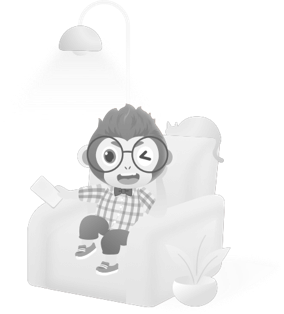# EE308_Lab4

 The Link My Class https://bbs.csdn.net/forums/MUEE308FZ The Link of Requirement of This Assignment https://bbs.csdn.net/topics/601188617 MU STU ID and FZU STU ID 19105754_831901114 Teammate's MU STU ID and FZU STU ID 19105819_831901130 Teammate's blog link https://bbs.csdn.net/topics/601429533 GitHub link https://github.com/Jodie-Rosie/Lab4_MIEC_Bobing Video demo link https://www.bilibili.com/video/BV1344y1x7CC?share_source=copy_web

🧠PSP form of this lab

💥Program Thinking

✍️Learning Progress Bar

💡Key Function of This Lab

🙋🙋‍♂️Teamwork with GitHub

📖Summary

🌃For Future

# 🧠PSP form of this lab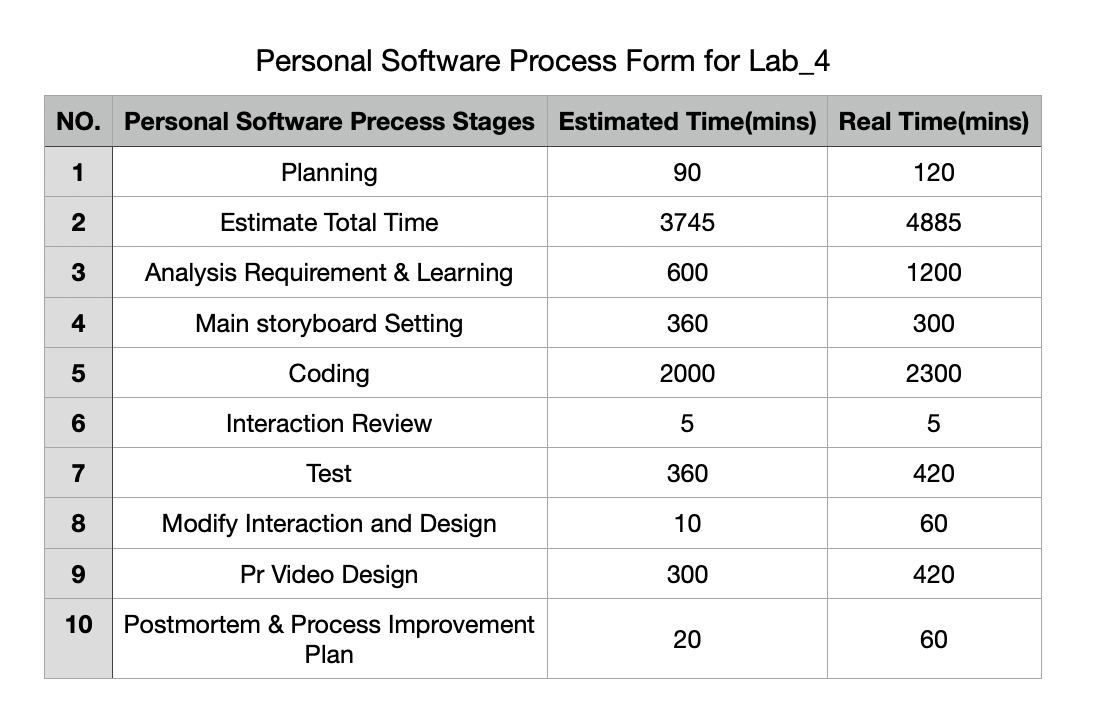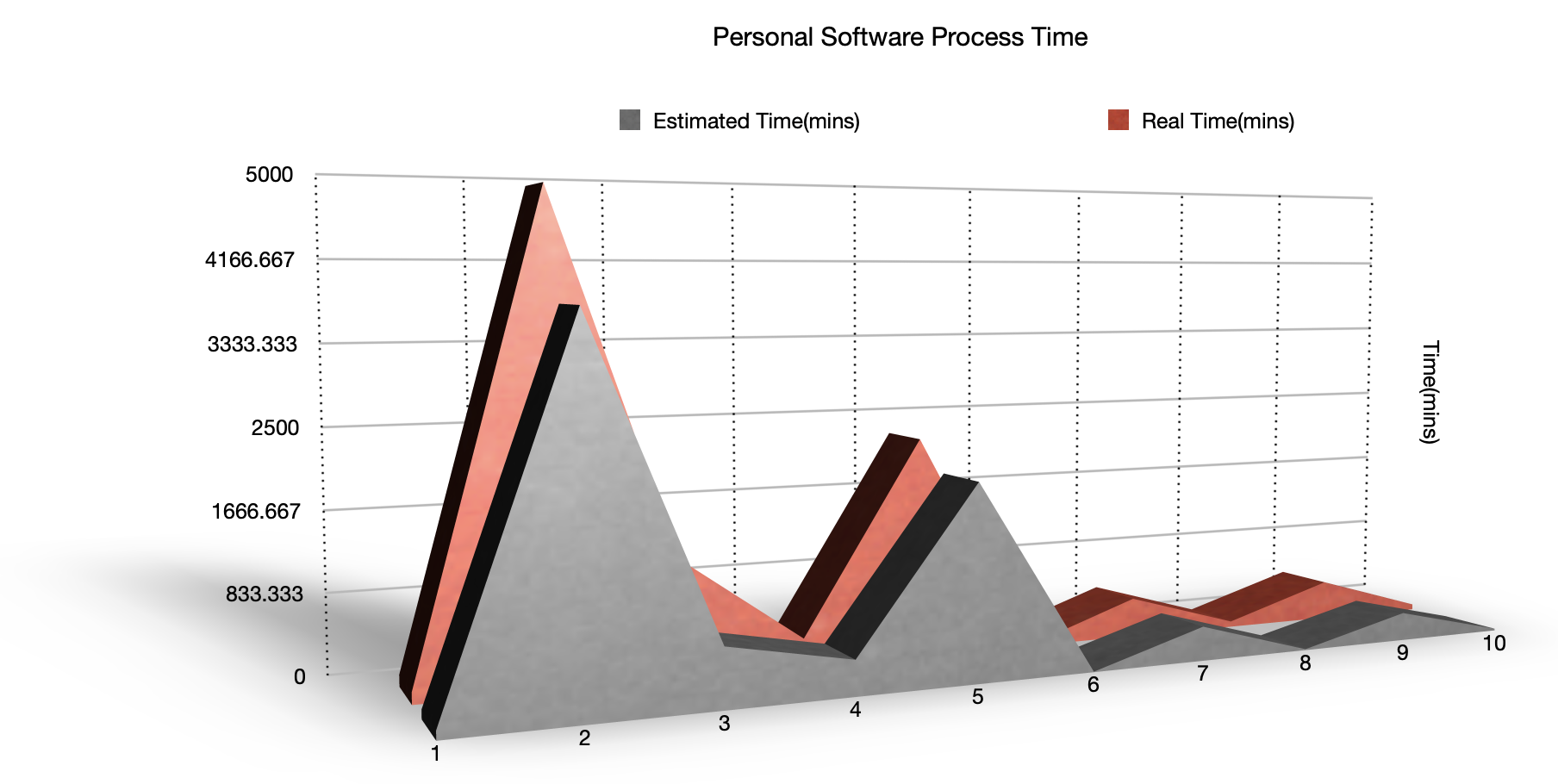# 💥Program Thinking

This is the first time we have completely written a software application. We should consider not only the front end and back end, but also the value transfer and interface problems, which are very difficult for us without a software foundation. And according to the UI design of our LAB 3, we decided to design the Bobing APP of the IOS system. By consulting a lot of materials, we decided to use Xcode compiler and Swift language for programming, which is friendly for beginners. For video making, we choose Adobe Pr and iMovie to make the video for our app.

Because both of us were new to Swift and App development, so we first studied swift language, and through some videos, blogs, and examples, let us understand the syntax and language style of swift. But we found that this is far from enough for us to implement UI design. We also used the open-source website to refer to other people's code and began to try to write it. Due to the tight time and heavy tasks, we have to compress our sleep and rest time.

In our lab, the more difficult and important aspects are:
1. Dice rotation and judgment of results.
2. Value transfer between pages.
3. Jump between button and page.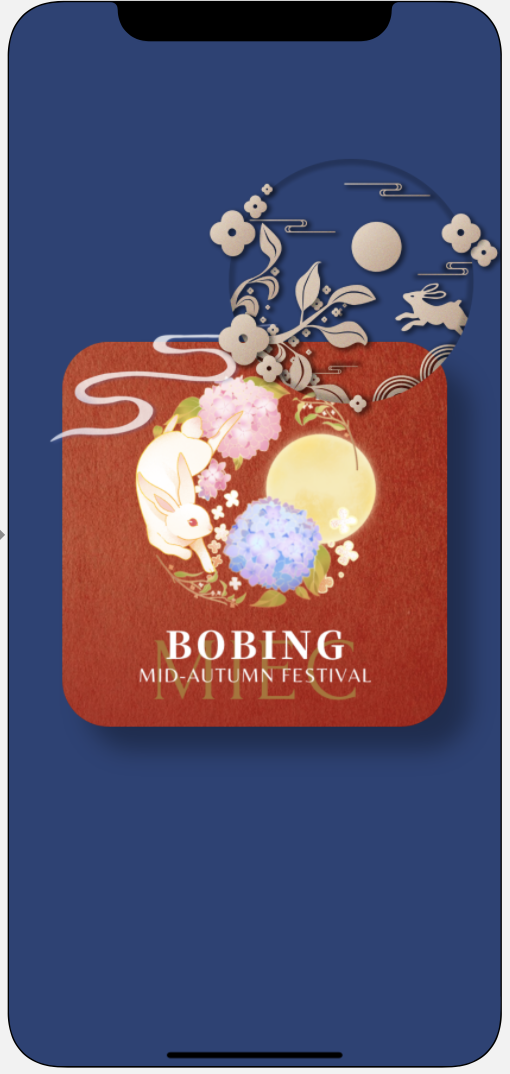# ✍️Learning Progress Bar

DayNew Coding LineAccumulated Coding LineElapsed Time(mins)Accumulated Elapsed Time(mins)What we have done
15050480480Learning how to code with Swift
2100150360840

Learning the basis of Xcode to develop an application

Finish the structure of the storyboard

33004504201260Coding the important part of View
45009505401800Coding important part of View and Learning the way to test our code
510010503602160Test the code and modify some bugs.
625013003602520Make App video and final test our program.

# 💡Key Function of This Lab

In order to realize the real-time output of blog cake results, we designed the following code:

I have thought about the relationship between the image in the Assets and the codes because we need to work out with 6 random values.

``````//
//  BobingINGViewController.swift
//  Lab4_Bobing_App
//
//  Created by Jodie Lin on 2021/10/7.
//

import UIKit

var ResultList = [UInt32]()
var someInts:[Int] = [0,1,2,3,4,5]
var Count_1:UInt32 = 0
var Count_2:UInt32 = 0
var Count_3:UInt32 = 0
var Count_4:UInt32 = 0
var Count_5:UInt32 = 0
var Count_6:UInt32 = 0
var Equal_6:UInt32 = 6
var Equal_5:UInt32 = 5
var Equal_4:UInt32 = 4
var Equal_3:UInt32 = 3
var Equal_2:UInt32 = 2
var Equal_1:UInt32 = 1
var Gname:String = String()

class BobingINGViewController: UIViewController {

@IBOutlet weak var Zhuangyuanlbl: UILabel!
@IBOutlet weak var BobingINGbackground: UIImageView!
@IBOutlet weak var Resultshowlbl: UILabel!
@IBOutlet weak var Resultdetaillbl: UILabel!
@IBOutlet weak var Resultbtn: UIButton!
@IBOutlet weak var RuleINGbtn: UIButton!
@IBOutlet weak var Rollnowbtn: UIButton!
@IBOutlet weak var Beforebtn: UIButton!
@IBOutlet weak var Groupnamelbl: UILabel!
@IBOutlet weak var Userimagebtn: UIButton!
@IBOutlet weak var Diceimage6: UIImageView!
@IBOutlet weak var Diceimage5: UIImageView!
@IBOutlet weak var Diceimage4: UIImageView!
@IBOutlet weak var Diceimage3: UIImageView!
@IBOutlet weak var Diceimage2: UIImageView!
@IBOutlet weak var Diceimage1: UIImageView!

var Gname:String = String()

let empty: String = String("Unnamed")

if Gname == ""
{
self.Groupnamelbl.text = empty
}
else{
self.Groupnamelbl.text = Gname

}
}

@IBAction func Rollnowbtn(_ sender: Any) {
let DiceNumber1 = arc4random_uniform(6) + 1
let DiceNumber2 = arc4random_uniform(6) + 1
let DiceNumber3 = arc4random_uniform(6) + 1
let DiceNumber4 = arc4random_uniform(6) + 1
let DiceNumber5 = arc4random_uniform(6) + 1
let DiceNumber6 = arc4random_uniform(6) + 1
ResultList.append(DiceNumber1)
ResultList.append(DiceNumber2)
ResultList.append(DiceNumber3)
ResultList.append(DiceNumber4)
ResultList.append(DiceNumber5)
ResultList.append(DiceNumber6)
for i in someInts{
if(ResultList[(i)] == Equal_1){
Count_1 = Count_1 + 1
}
if(ResultList[(i)] == Equal_2){
Count_2 = Count_2 + 1
}
if(ResultList[(i)] == Equal_3){
Count_3 = Count_3 + 1
}
if(ResultList[(i)] == Equal_4){
Count_4 = Count_4 + 1
}
if(ResultList[(i)] == Equal_5){
Count_5 = Count_5 + 1
}
if(ResultList[(i)] == Equal_6){
Count_6 = Count_6 + 1
}
}

if Count_1 == Equal_2 && Count_4 == Equal_4
{
Resultshowlbl.text = "Zhuangyuan"
Zhuangyuanlbl.text = "Gold Flower"
Count_1 = 0
Count_2 = 0
Count_3 = 0
Count_4 = 0
Count_5 = 0
Count_6 = 0
}
else if Count_4 == Equal_6
{
Resultshowlbl.text = "Zhuangyuan"
Zhuangyuanlbl.text = "Six Cup of Red"
Count_1 = 0
Count_2 = 0
Count_3 = 0
Count_4 = 0
Count_5 = 0
Count_6 = 0
}
else if Count_6 == Equal_6
{
Resultshowlbl.text = "Zhuangyuan"
Zhuangyuanlbl.text = "Six Cups of Black"
Count_1 = 0
Count_2 = 0
Count_3 = 0
Count_4 = 0
Count_5 = 0
Count_6 = 0
}
else if Count_4 == Equal_5
{
Resultshowlbl.text = "Zhuangyuan with Five Red"
Zhuangyuanlbl.text = "  "
Count_1 = 0
Count_2 = 0
Count_3 = 0
Count_4 = 0
Count_5 = 0
Count_6 = 0
}
else if Count_3 == Equal_5
{
Resultshowlbl.text = "Zhuangyuan with WuZiDengKe"
Zhuangyuanlbl.text = "  "
Count_1 = 0
Count_2 = 0
Count_3 = 0
Count_4 = 0
Count_5 = 0
Count_6 = 0
}
else if Count_4 == Equal_4
{
Resultshowlbl.text = "Zhuangyuan with Four Points"
Zhuangyuanlbl.text = "  "
Count_1 = 0
Count_2 = 0
Count_3 = 0
Count_4 = 0
Count_5 = 0
Count_6 = 0
}
else if Count_1 == Equal_1 && Count_2 == Equal_1 && Count_3 == Equal_1 && Count_4 == Equal_1 && Count_5 == Equal_1
{
Resultshowlbl.text = "Dui Tang"
Zhuangyuanlbl.text = "  "
Count_1 = 0
Count_2 = 0
Count_3 = 0
Count_4 = 0
Count_5 = 0
Count_6 = 0
}
else if Count_4 == Equal_3
{
Resultshowlbl.text = "Three Red"
Zhuangyuanlbl.text = "  "
Count_1 = 0
Count_2 = 0
Count_3 = 0
Count_4 = 0
Count_5 = 0
Count_6 = 0
}
else if Count_2 == Equal_4
{
Resultshowlbl.text = "Four Jin"
Zhuangyuanlbl.text = "  "
Count_1 = 0
Count_2 = 0
Count_3 = 0
Count_4 = 0
Count_5 = 0
Count_6 = 0
}
else if Count_4 == Equal_2 && Count_2 != Equal_4
{
Resultshowlbl.text = "Two Ju"
Zhuangyuanlbl.text = "  "
Count_1 = 0
Count_2 = 0
Count_3 = 0
Count_4 = 0
Count_5 = 0
Count_6 = 0
}
else if Count_4 == Equal_1
{
Resultshowlbl.text = "One Xiu"
Zhuangyuanlbl.text = "  "
Count_1 = 0
Count_2 = 0
Count_3 = 0
Count_4 = 0
Count_5 = 0
Count_6 = 0
}
else{
Resultshowlbl.text = "Thanks"
Zhuangyuanlbl.text = "  "
Count_1 = 0
Count_2 = 0
Count_3 = 0
Count_4 = 0
Count_5 = 0
Count_6 = 0
}
ResultList = [UInt32]()
Diceimage1.image = UIImage(named: "Dice\(DiceNumber1)")
Diceimage2.image = UIImage(named: "Dice\(DiceNumber2)")
Diceimage3.image = UIImage(named: "Dice\(DiceNumber3)")
Diceimage4.image = UIImage(named: "Dice\(DiceNumber4)")
Diceimage5.image = UIImage(named: "Dice\(DiceNumber5)")
Diceimage6.image = UIImage(named: "Dice\(DiceNumber6)")

}

@IBAction func RuleINGbtn(_ sender: Any) {
self.performSegue(withIdentifier:"GRtoRules", sender: self)
}

@IBAction func Resultbtn(_ sender: Any) {
self.performSegue(withIdentifier:"INGtoResult", sender: self)
}
@IBAction func BeforetoHome(_ sender: Any) {
self.performSegue(withIdentifier:"INGtoHome", sender: self)
}``````

After Coding, we are able to see the result: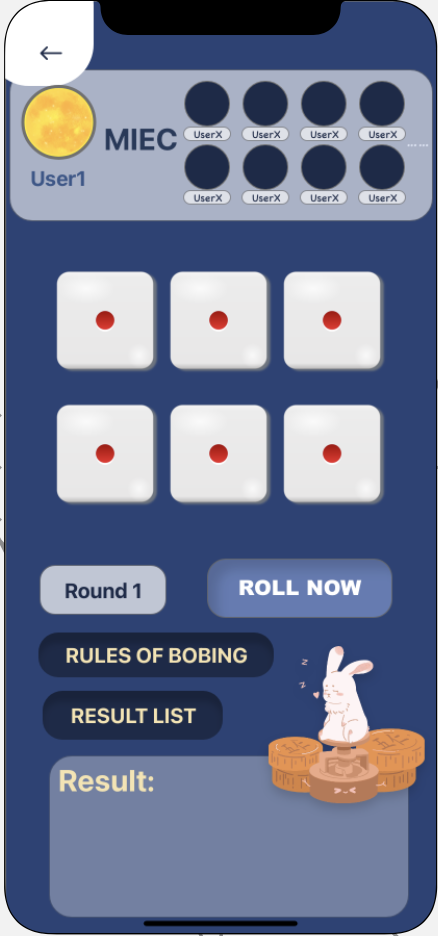For realizing the action that if users click the button, the page will change, we write the code:

``````//
//  ViewController.swift
//  Lab4_Bobing_App
//
//  Created by Jodie Lin on 2021/10/6.
//
import UIKit
class ViewController: UIViewController {
@IBOutlet weak var Nextbtn: UIButton!
@IBOutlet weak var Beforeallpage: UIImageView!

}

@IBAction func NEXTbtn(_ sender: Any) {
self.performSegue(withIdentifier:"next", sender: self)
}
}
``````

Then we use the segue to share values between different pages:

``````// MARK: - Navigation

// In a storyboard-based application, you will often want to do a little preparation before navigation
override func prepare(for segue: UIStoryboardSegue, sender: Any?) {
if segue.destination is ProfileViewController
{
let VC = segue.destination as? ProfileViewController

VC?.Location = self.Locationlbl.text!
VC?.Email = self.Emaillbl.text!
VC?.Company = self.Grouplbl.text!
VC?.Birthday = self.Birthdaylbl.text!
VC?.Gender = self.Genderlbl.text!
VC?.UserID = self.IDlbl.text!
}

// Get the new view controller using segue.destination.
// Pass the selected object to the new view controller.
}``````

# 🙋🙋‍♂️Teamwork in This Lab

At first, Rosie and I just discuss through Wechat and start the program on my GitHub from the time that I begin to learn Swift and developing IOS in Xcode. So both of us don't know much about the GitHub organization using. Here is my original personal GitHub page link (Which we copy to the new one):

https://github.com/Jodie-Rosie/Lab4_Bobing_App/commits/main

Then we found out that we can use GitHub organization to share our code, which helps us a lot when the time that we were coding.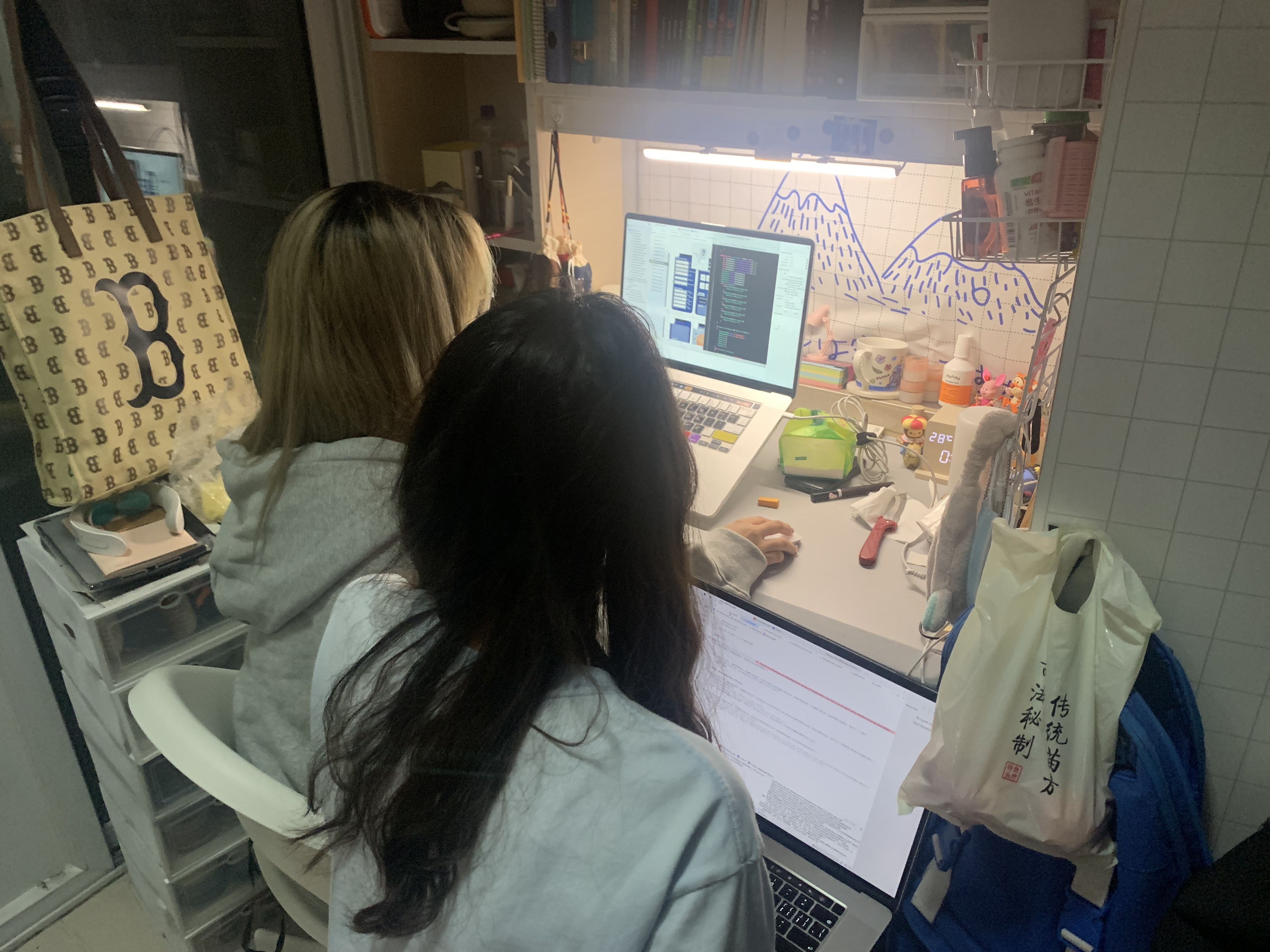# 📖Summary

From this lab, both of us have the brand-new cognition to our major Electronic Engineering, we can't believe in just two weeks we had learned to zero basis to UI design and to the final App implementation, on the way we also want to give up, because of the difficulty of making App really is more than others to make web pages or small programs, but we finally persevered. At the beginning, my teammates and I made different progress in the experiment due to different computer systems. I first tried the OS system to develop Xcode, while my teammate could not use Swift language to develop simultaneously with me because she was a Windows computer. Finally, she borrowed a Mac computer. We've just officially started our software development. We tried to use it online in the past, but we had to give up the learning and development of this part because the connection between App and wechat sharing interface requires registration and audit team, and we need to provide the link of App listing and the homepage of the company, which we lack. We did our best to make this experiment as good as we could.

# 🌃For Future

in the future, I decide to learn more knowledge about the development of app through OS system, which is because through this LAB, I find it is interesting. Also, I will try my best to deal with the following lab, even though EE308 is almost full of my life now....全文
94 点赞 收藏 回复